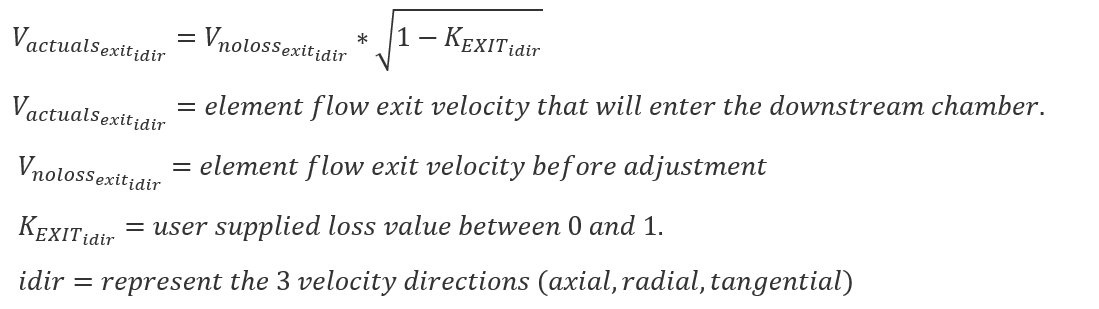# Element Exit K Loss Corrections

Most Flow Simulator elements can calculate a velocity of the flow exiting the element. This flow velocity is a part of the flow dynamic head and momentum. All elements entering a chamber contribute to the magnitude and direction of the flow velocity in the downstream chamber. Users can adjust the magnitude of the velocity in 3 directions using the Exit K Loss input.

Most elements will not need an adjustment to the exit velocity using the inputs described here. It is better to rely on proper element orientation inputs and proper flow resistance inputs.

## Exit K Loss in the GUI

The user input for the Exit K Loss can be found in the advanced options section of restrictive type elements.None=Do not reduce exit velocity. (default)

Specify K Loss = Enter a loss value between 0 (no loss) and 1 (all lost, exit velocity = 0) for each of the 3 directions.

## Exit K Loss Theory

The element exit velocities are adjusted using this equation:## Related Element Inputs

The user entry is labeled K_EXIT_Z (U and R) in the database (.flo) file.

 Element Input Variables Index UI Name (.flo label) Description Varies with Element Exit K Loss: Axial (K_EXIT_Z) Tangential (K_EXIT_U) Radial (K_EXIT_R) Head loss factors in the Z, U, and R directions based on the spherical coordinate system of theta and phi. Z theta and phi equal zero (the axial direction in a rotational field). U theta equals 90 and phi equals zero (the tangential direction in a rotational field). R theta equals zero and phi equal 90 zero (the radial direction in a rotational field). Valid values of K_EXIT_i (i = Z, U, R) range from zero (default) to one The three loss factors reduce the corresponding three components of velocity (idir) exiting the element(Default value, 0.0, provides no loss)

## Related Element Outputs

Outputs in file with “res” extension. Output units controlled by user setting in “Output Control” panel.

Name Description Units ENG, SI
K_EXIT (Z, R, U) User Inputs for the exit dynamic head loss. none
VAXIAL, VRAD, VTAN_ABS Element E\exit velocities in the axial, radial, and tangential direction.

ft/sec,

m/sec